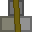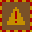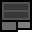# Account 803203

{C6F7} Hunter's DredBank
Transfer Bank

## BalanceMetal: 0s2 = 2Explosives: 93s2 = 1490RC Turrets: 35

## Transfer

 PIN Receiver ReferenceMetal 16 × +Explosives 16 × +RC Turrets

## Create new account token

PIN:

New accounts cost 2 stacks (32 units) metal. You must give the token directly to the other player. Do not create the account yourself and give them the details. If you are found to do this, your account will be closed and all your items taken. You are responsible for ensuring the player you provide a new account token to knows how to use dredbank properly. If many players are found who don't know how to use dredbank properly, and they all got their accounts from one player, there may be a punishment against the player who provided their accounts.

## Transactions

Type Counterparty Account Reference NumberMetalExplosivesRC Turrets
Sent 220022 666 39s0 = 624 0s0 = 0 0
Received 396705 777 0s0 = 0 50s0 = 800 0
Sent 321000 0 2s0 = 32 0s0 = 0 0
Received 286992 1290 20s0 = 320 0s0 = 0 0
Sent 321000 0 2s0 = 32 0s0 = 0 0
Received 286992 5678 0s0 = 0 0s0 = 0 10
Received 286992 5678 0s0 = 0 0s0 = 0 10
Received 286992 1209 10s0 = 160 10s0 = 160 0
Received 694228 5732 11s0 = 176 0s0 = 0 0
Sent 286992 1234 50s0 = 800 23s0 = 368 0
Sent 286992 1234 0s0 = 0 0s0 = 0 10
Sent 286992 1234 0s0 = 0 0s0 = 0 10
Sent 321000 0 2s0 = 32 0s0 = 0 0
Sent 321000 0 2s0 = 32 0s0 = 0 0
Sent 694228 0 11s0 = 176 0s0 = 0 0
Sent 321000 0 2s0 = 32 0s0 = 0 0
Received 808201 3456 16s0 = 256 16s0 = 256 0
Received 808201 3456 16s0 = 256 16s0 = 256 0
Sent 321000 1234 0s0 = 0 0s0 = 0 1
Sent 220022 6644 0s0 = 0 0s0 = 0 36
Sent 220022 6644 0s0 = 0 23s0 = 368 0
Sent 808201 0 0s0 = 0 16s0 = 256 0
Sent 220022 6644 36s0 = 576 0s0 = 0 0
Sent 837562 0 0s0 = 0 0s0 = 0 20
Received 151732 1 0s0 = 0 0s0 = 0 1
Sent 151732 0 0s0 = 0 0s0 = 0 1
Sent 220022 13 10s0 = 160 0s0 = 0 0
Sent 220022 12 10s0 = 160 0s0 = 0 0
Deposit 0 0s0 = 0 32s2 = 514 0
Deposit 0 0s0 = 0 0s0 = 0 19
Deposit 0 3s6 = 54 0s0 = 0 0
Deposit 0 10s12 = 172 0s0 = 0 0
Deposit 0 79s0 = 1264 31s0 = 496 1
Deposit 0 0s0 = 0 0s0 = 0 43
Received 151732 1 0s0 = 0 0s0 = 0 1
Sent 151732 1 0s0 = 0 0s0 = 0 1
Deposit 0 0s0 = 0 0s0 = 0 25
Deposit 0 0s0 = 0 0s0 = 0 4